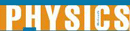# Moving Objects and Velocity - Research Article from World of Physics

This encyclopedia article consists of approximately 3 pages of information about Moving Objects and Velocity.
 This section contains 781 words (approx. 3 pages at 300 words per page) View a FREE sampleWhen an object moves, its position changes as a function of time, which is the definition of displacement. The distance (d) of the displacement is a scalar quantity and the displacement (d) of the object is a vector quantity, i.e. it has both a magnitude and a direction. If at time t the object is at position P and if it is displaced to position P at a time t, the displacement, d in the time interval t = t- t, is equal to P-P. The average velocity during the displacement is equal to v = d/t, another vector quantity. The distance traveled during the displacement divided by the time interval of the displacement is the average speed of the object, v = d/t, a scalar quantity. More useful when studying moving objects however, are the concepts of instantaneous velocity and speed...

 This section contains 781 words (approx. 3 pages at 300 words per page) View a FREE sample